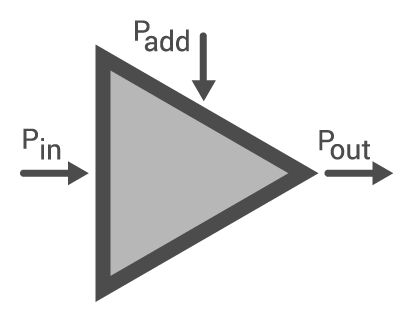## A calculator designed to compute the power-added efficiency (PAE) of an amplifier

(%)

### Overview

An amplifier is able to amplify an input signal because of the added power by its DC source. Power-added efficiency is a convenient parameter that is used to determine how much the DC input power contributes to the amplification of an input signal.

This tool is designed to calculate the power-added efficiency given three values: input power, output power and DC power. To use this, simply place the required values and click the "calculate" button. Take note that you can select between milliwatts, watts, decibel-milliwats (dBm) or decibel-watts (dbW) for the units of the input power, output power and DC power.### Equations

$$Efficiency_{\text{power added}} = \frac{P_{out}-P_{in}}{P_{add}} \text{ x }100$$

Where:

$$P_{in}$$ = input power

$$P_{out}$$ = output power

$$P_{add}$$ = dc added power

### Applications

Efficiency is used to calculate how much input power a device converts into useful output power. A device which has an efficiency of 100% means that all its input power is converted into output power. Of course, no such device exists. Nevertheless, engineers use efficiency calculations to determine how much power is converted to waste power, which in most cases, is heat.

In the case of amplifiers, efficiency is the ratio between the output power to the DC input power. This is known as drain efficiency. However this type of efficiency can be misleading to Engineers as it does not consider how much power is utilized by the amplifier. An amplifier can have a high drain efficiency and at the same time have a very low gain. This is why most Engineers, especially RF Engineers, use power-added efficiency instead. With power-added efficiency, the input power is now considered (see equation above). Most of the time, a device with a high power-added efficiency is preferred over a device with high drain efficiency.